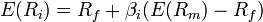×

# CAPM Calculator

Fill in any three to calculate the fourth value:
 Expected return on the capital asset (E(Ri)): % Risk free rate of interest (Rf): % Expected return of the market (E(Rm)): % Beta for capital asset (βi):

The CAPM Calculator is used to perform calculations based upon the capital asset pricing model. It will calculate any one of the values from the other three in the CAPM formula.

## CAPM (Capital Asset Pricing Model)

In finance, the CAPM (capital asset pricing model) is a theory of the relationship between the risk of a security or a portfolio of securities and the expected rate of return that is commensurate with that risk. The theory is based on the assumption that security markets are efficient and dominated by risk averse investors. risk averse investors. It is used to determine a theoretically appropriate required rate of return of an asset, if that asset is to be added to an already well-diversified portfolio, given that asset's non-diversifiable risk.

## Formula

The measurable relationship between risk and expected return in the CAPM is summarized by the following formula:where:
E(Ri) = the expected return on the capital asset
Rf = the risk-free rate of interest such as a U.S. Treasury bond
βi = the beta of security or portfolio i
E(Rm) = the expected return of the market

## References

1. http://en.wikipedia.org/wiki/Capital_asset_pricing_model

## All of Our Miniwebtools (Sorted by Name):

•{{name}}
{{title}}

Watch Videos: